# AFOQT: Lines & Angles Chapter Exam

Exam Instructions:

Choose your answers to the questions and click 'Next' to see the next set of questions. You can skip questions if you would like and come back to them later with the yellow "Go To First Skipped Question" button. When you have completed the practice exam, a green submit button will appear. Click it to see your results. Good luck!

### Page 2

#### Question 6 6. If angle 1 measures 120, what must angle 5 equal if the lines are parallel?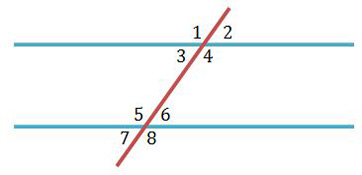#### Question 9 9. Which angle is equal to angle 1?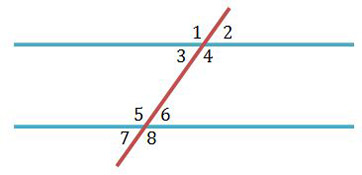### Page 3

#### Question 12 12. What must angle 8 equal if angle 1 measures 120 degrees and you wanted to prove the lines parallel?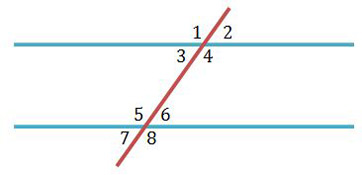#### Question 13 13. What is the distance of this line segment?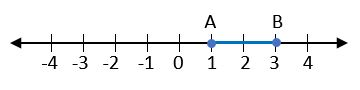#### Question 14 14. Name a pair of corresponding angles.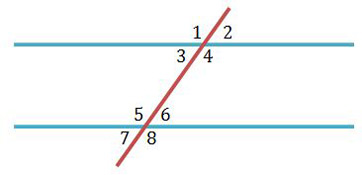#### Question 15 15. Name a pair of vertical angles.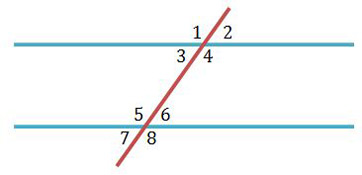### Page 4

#### Question 18 18. Which line is the transversal?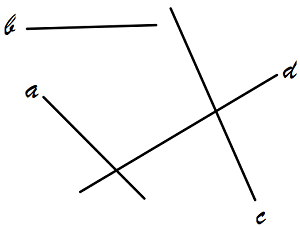#### Question 19 19. To prove the lines are parallel, if angle 3 measured 30 degrees, what must angle 6 measure?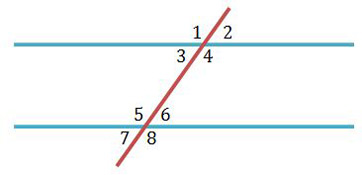### Page 5

#### Question 21 21. If angle 3 and 5 added up to 180, which converse statement can you use to prove the lines are parallel?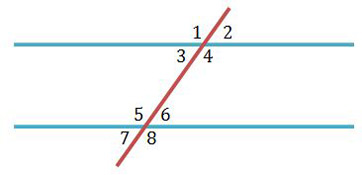#### Question 22 22. Consider the figure below, where Angle 4 = 12m - 4 Angle 5 = 10m + 20 Calculate the measure of Angle 5.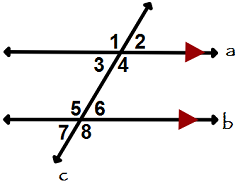#### Question 23 23. Given the figure below, Angle 3 = 140y and Angle 5 = 80y + 70. Determine the value of y.### Page 6

#### Question 27 27. If angle 2 measures 60, what does angle 8 measure if the lines are parallel?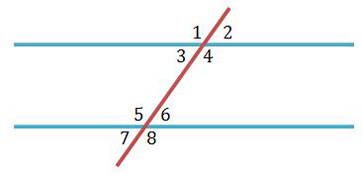#### Question 28 28. Which angle is the alternate exterior angle to angle 2?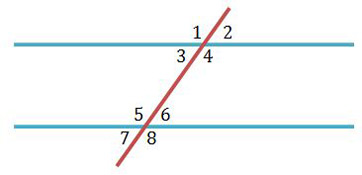#### Question 29 29. What is the relationship between Angle 4 and Angle 1?#### Question 30 30. What is the relationship between Angle 1 and Angle 7?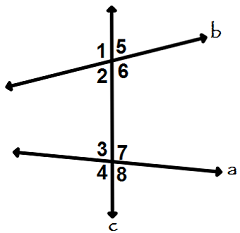#### AFOQT: Lines & Angles Chapter Exam Instructions

Choose your answers to the questions and click 'Next' to see the next set of questions. You can skip questions if you would like and come back to them later with the yellow "Go To First Skipped Question" button. When you have completed the practice exam, a green submit button will appear. Click it to see your results. Good luck!

Support